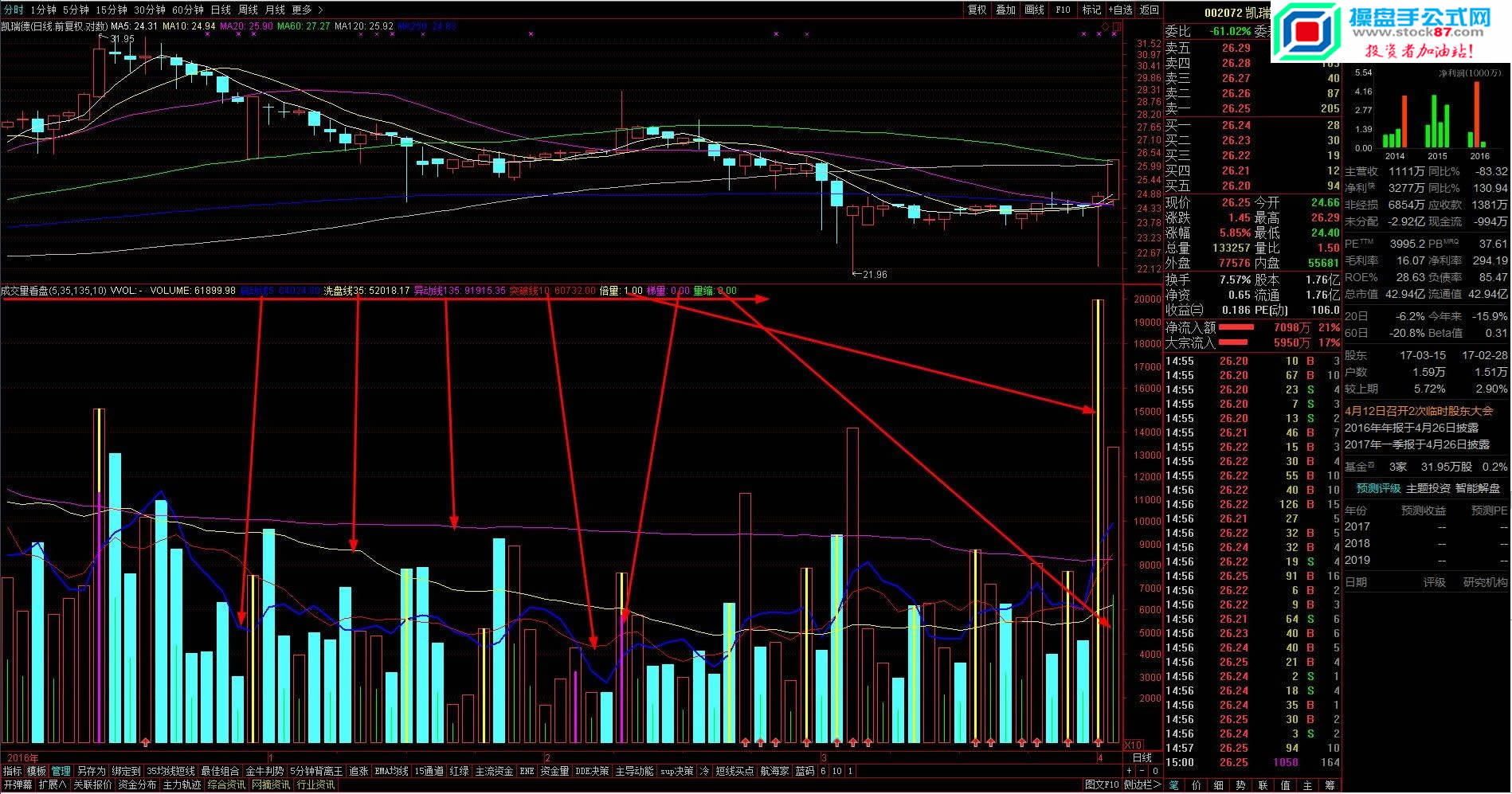﻿ 成交量看盘（源码 副图 通达信 贴图）也许对你有用-vol-操盘手公式网当前位置：首页 >> 股票公式 >>  vol

• 相关简介： 今日股市
• 内容标签： 成交量,通达信
• 浏览次数：
• 运行环境： Win10,Win8,Win7,WinXP
• 文章作者： 操盘手公式网
• 文章来源： www.stock87.com
• 加入时间： 2020-04-07 11:35:49
• 解压密码： www.stock87.com（请仔细输入！复制无效！）
•TOTAL:=IF(PERIOD=1,5,IF(PERIOD=2,15,IF(PERIOD=3,30,IF(PERIOD=4,60,IF(PERIOD=5,TOTALFZNUM,1)))));
MTIME:=MOD(FROMOPEN,TOTAL);
CTIME:=IF(MTIME<0.5,TOTAL,MTIME);
VVOL:IF((CURRBARSCOUNT=1 AND DYNAINFO(8)>1),VOL*TOTAL/CTIME,DRAWNULL),NODRAW;
STICKLINE((CURRBARSCOUNT=1 AND DYNAINFO(8)>1),VVOL,0,-1,-1),COLOR00C0C0;
VOLUME:VOL,VOLSTICK;
X_1:=MA(VOLUME,M1);
X_2:=MA(VOLUME,M2);
X_3:=MA(VOLUME,M3);
X_7:=MA(VOLUME,M4);

STICKLINE(倍量,0,VOL,0.3,0),COLORYELLOW;
STICKLINE(梯量,0,VOL*0.75,0.3,0),COLORMAGENTA;
STICKLINE(量缩,0,VOL*0.5,0,0),COLORGREEN;
X_4:=BARSLASTCOUNT(X_1>X_2 AND X_1>REF(X_1,1) AND X_1<=X_3);
X_5:=X_4>0;
X_6:=CROSS(X_1,X_3) AND X_3>X_2;
DRAWICON(X_5 OR X_6,0,1);### 热搜标签浙ICP备17017803号-1